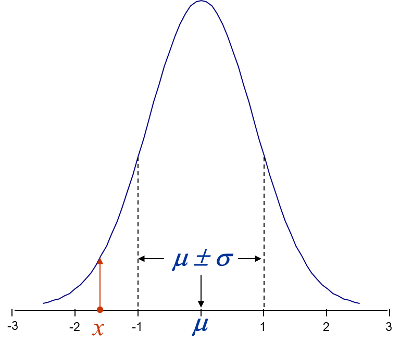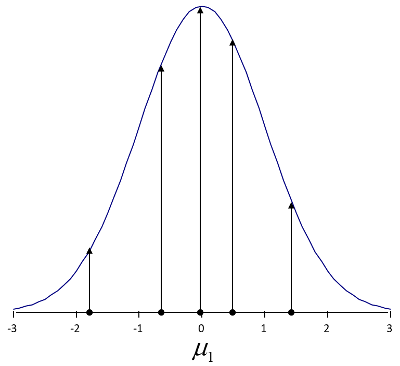## Probability and Likelihood

### Probability:###### Figure 1 – Probability density showing the probability that an observation will fall between two given values of $$x$$. The red ordinate shows the (relative) probability of a single observation.

• The probability density function, pdf, tells how probable a value of $$x$$ is, given the model parameters, $$\theta$$. For example, for a Normal density: $$\theta= (\mu, \sigma)^T$$$f(x | \mu, \sigma) = \frac{1}{\sqrt{2 \pi}} e^{-\frac12 \left(\frac{x-\mu}{\sigma} \right)^2 }$
• Probabilities integrate to one.

### Likelihood:###### Figure 2 – Likelihood function showing the likelihood of the parameter values, $$\theta= (\mu, \sigma)^T$$, given the observations,.

$f(\mu, \sigma | x) = \frac{1}{\sqrt{2 \pi}} e^{-\frac12 \left(\frac{x-\mu}{\sigma} \right)^2 }$(Notice that the likelihood and probability formulations are identical; the only difference is in what is known: probability (i.e. the model parameters) or observed results, $$x$$.)

• The likelihood function tells how likely the model parameters, $$\theta= (\mu, \sigma)^T$$ are, given the observed value of $$x$$.
• Likelihood can be defined for both censored and uncensored data.(Uncensored example shown here.)
• Likelihoods do not necessarily integrate to one.